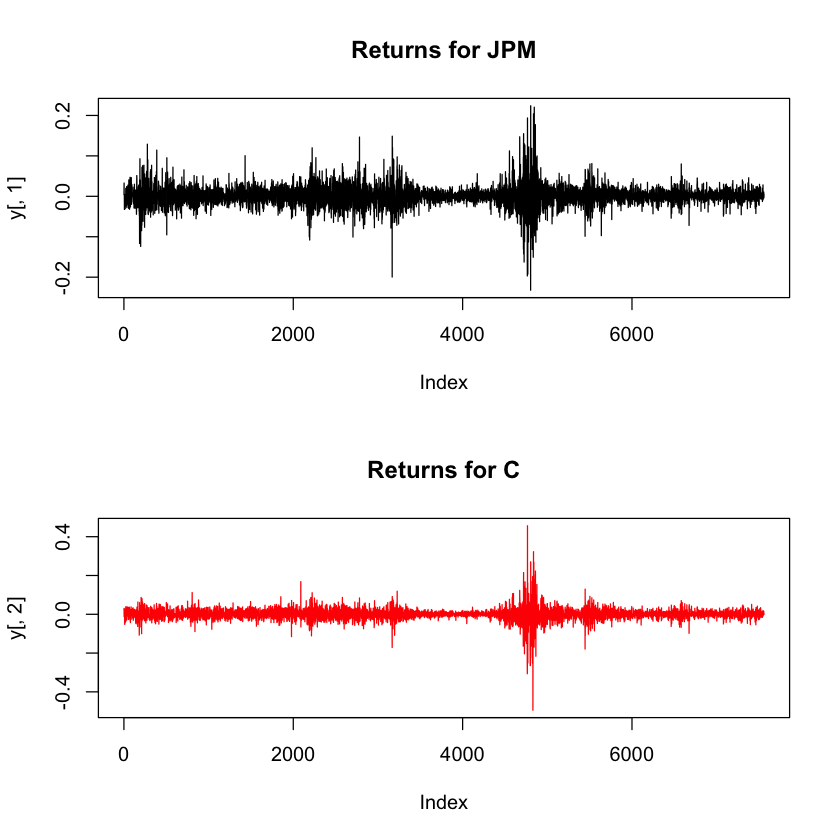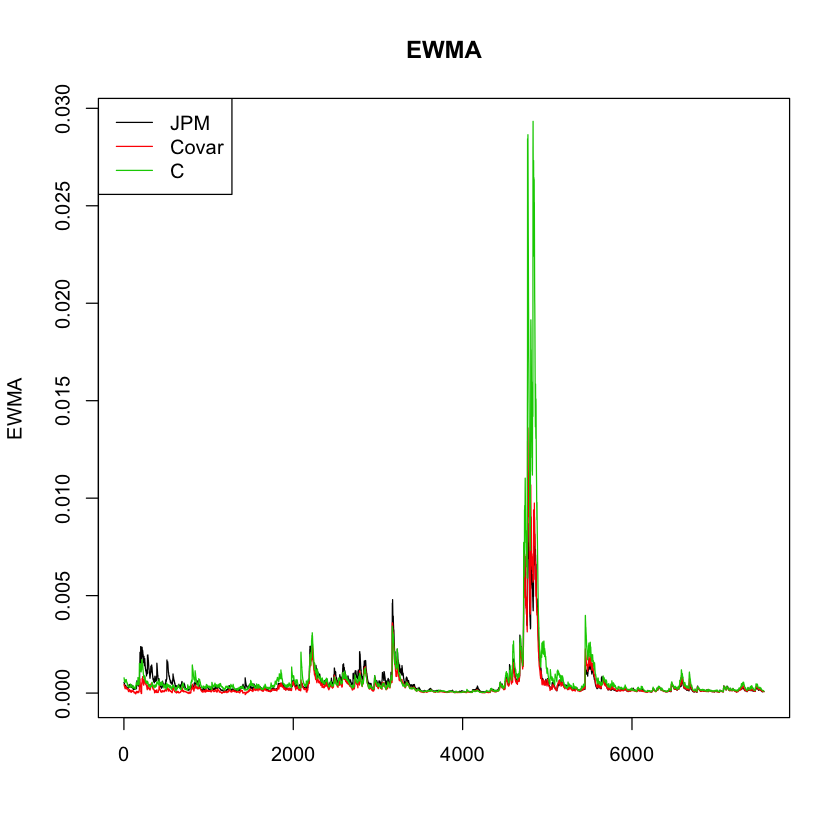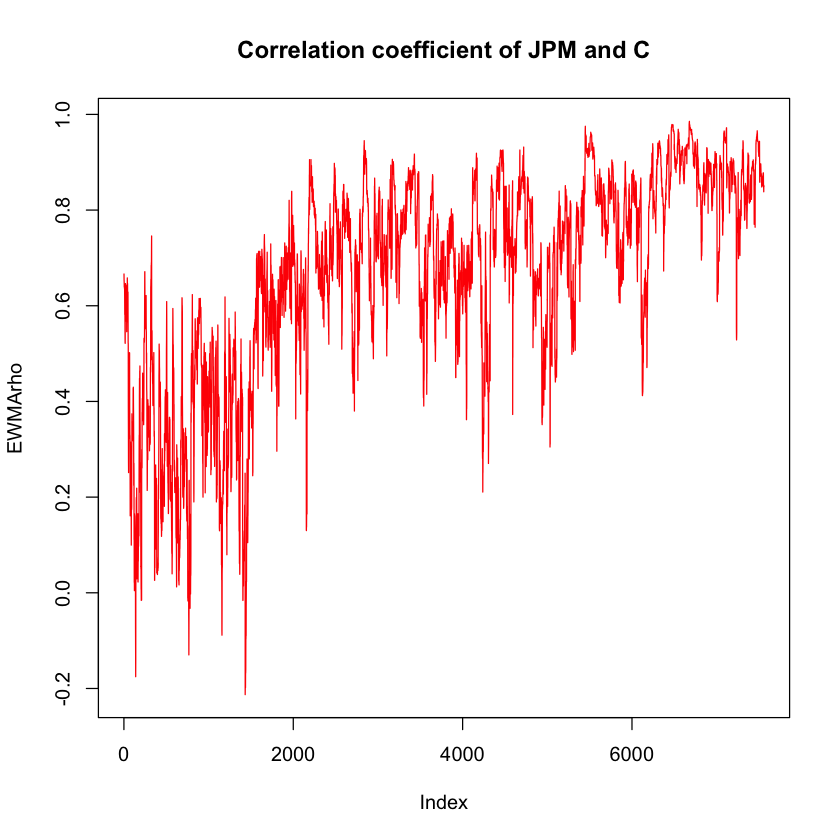# Seminar 5

In this class we will:

We first load the packages for this seminar:

In :
library(rmgarch)
library(microbenchmark)
library(lubridate)


## Multivariate volatility models¶

In a univariate volatility model, we consider a stock with returns $Y_t$ that can be written as:

$$Y_t = \sigma_{t} Z_{t}$$

Where $\sigma_t$ is the conditional volatility and $Z_t$ are random shocks.

For many financial applications, we have to consider a vector of assets, instead of a single asset. So, if we have $K>1$ assets, it is necessary to indeicate which asset and paremeters are being referred to. The notation we will use is:

$$Y_{t,i} = \sigma_{t,i} Z_{t,i}$$

Where the first subscript indicates the date, and the second subscript the asset.

Now our conditional covariance is a symmetric square conditional covariance matrix, where the dimension is the number of assets. For example, for three assets it would be:

$$\begin{equation*} \Sigma_{t} = \begin{pmatrix} \sigma_{t,11} & & \\ \sigma_{t,12} & \sigma_{t,22} & \\ \sigma_{t,13} & \sigma_{t,23} & \sigma_{t,33} \end{pmatrix} \end{equation*}$$

If we have a portfolio wight a vector $w$ of portfolio weights, then the portfolio variance would be:

$$\sigma^2_{\textrm{portfolio}} = w'\Sigma w$$

As in the case of univariate models, we need to ensure that the variance is not negative. In this case, this means that the covariance matrix $\Sigma$ is positive semi-definite:

$$|\Sigma| \geq 0$$

Working with several assets presents the curse of dimensionality. This means that the number of variance and covariance terms will explode as the number of assets increase, which makes it challenging to estimate the covariance matrix and ensure its positive semi-definiteness.

For example, if we try to estimate a GARCH model for two assets, we would have 21 parameters to estimate, which is almost impossible in practice.

The concept of stationarity is more important in multivariate volatility models, since violating it could lead to numerical problems. In this seminar we will focus in implementing three different multivariate models:

• Exponentially-Weighted Moving Average
• Dynamic Conditional Correlation Models
• Orthogonal-GARCH

To do so, we will work with the returns from JP Morgan and Citigroup.

In :
# Load the data

# Extract the returns for JPM and C
y <- Y[c("JPM", "C")]


## EWMA¶

In the Exponentially-Weighted Moving Average model, the estimated conditional volatility at time $t$ is a convex combination of the estimation at time $t-1$ and the squared returns at time $t-1$. In general, if we have a vector of returns for each time $t$ that includes all assets $K$:

$$\textbf{y}_{t} = [y_{t,1}, y_{t,2}, \ldots, y_{t,K}]$$

Then the multivariate EWMA is:

$$\hat{\Sigma}_{t} = \lambda \hat{\Sigma}_{t-1} + (1-\lambda) \textbf{y}'_{t-1} \textbf{y}_{t-1}$$

The properties of this mdoel are:

• The same pre-specified weight, $\lambda$ is used for all assets
• The variance of any particular asset only depends on its own lags

### Implementation¶

Since we will perform linear algebra operations to compute the EWMA, it is more convenient to work with a matrix instead of a dataframe, so let's first turn y from a dataframe into a matrix:

In :
# Check the class of y
class(y)

# Transform into matrix
y  <- as.matrix(y)

# Check class again
class(y)

'data.frame'
'matrix'

Let's take a look at the returns:

In :
# Plotting the returns in a 2x1 grid
par(mfrow = c(2,1))
plot(y[,1], type = "l", main = "Returns for JPM", col = 1)
plot(y[,2], type = "l", main = "Returns for C", col = 2)
# Reset the grid
par(mfrow = c(1,1))We will create a EWMA matrix that will hold our estimations for the elements of $\Sigma$. First let's establish the number of entries the matrix should have:

In :
# Determine number of entries
n <- dim(y)


Now we create the matrix. We could initialize the matrix with a number, like 0, but it is recommended to initialize it with NA to avoid any potential mistakes.

To determine the number of columns in the matrix, we need to figure out how many parameters we are estimating in every time period. For any given time $t$, we will be estimating the conditional variance of each asset, and the conditional covariances. For $K$ assets, this is:

$$K + K(K-1)/2$$

In our case, it is 3. Column 1 will hold the conditional variance of JPM, column 2 will hold the conditional covariance between JPM and C, and column 3 will hold the conditional variance for Citigroup:

In :
# Initializing the EWMA matrix
EWMA <- matrix(NA, nrow = n, ncol = 3)


#### Why do we initialize a matrix/vector?¶

It is convenient to create the vector or matrix we want to populate before doing so for two reasons:

1. Good programming practice. It is better to have a fixed-length object than to re-size an object in every iteration of the loop.
2. Be able to spot mistakes. In case there is an error in your loop and try to allocate an element to a non-existing row, you will get an error message. If you are dynamically modifying the size of the object, you can add more rows/columns by accident without realizing it

We could fill the new matrix with any values, and it is common to fill them with zeros. However, it is useful to fill the matrix with NA. This way, if something in the loop doesn't work, out matrix will have some empty spots instead of holding zeros, and whenever we try to make any operation we will get an error message.

We determine the value for $\lambda$:

In :
# Determine lambda
lambda <- 0.94


A common problem is to determine how to estimate the conditional covariances of the first period. In other words, how do we estimate $\hat{\Sigma}_1$ without $\hat{\Sigma}_0$ or $\textbf{y}_0$. For this, we will use the unconditional covariance of the sample and "burn" the first few observations. The effect of a given conditional covariance from a past period quickly dies out as time passes, because $\lambda^n \rightarrow 0$ as $n \rightarrow \infty$, so the effect of initializing the EWMA matrix with the unconditional covariance will not be a problem after a few time periods. A rule of thumb is to burn the first 30 observations.

In :
# Get the sample covariance
S <- cov(y)
S

JPMC
JPM0.00054877140.0004345622
C0.00043456220.0007748630

We want to get the three unique values of the matrix. This can be done in three different ways shown below. We recommend using the last, since it is easily reproducible for any number of assets:

In :
# Getting the unique values in three ways:

# 1. Creating a vector with the distinct elements
c(S[1,1], S[1,2], S[2,2])

# 2. Vectorizing the matrix and getting distinct elements
c(S)[c(1,2,4)]

# 3. Using the fact that S is symmetric and using upper.tri()/lower.tri()
S[!upper.tri(S)]

1. 0.000548771444357849
2. 0.000434562198194613
3. 0.00077486302054072
1. 0.000548771444357849
2. 0.000434562198194613
3. 0.00077486302054072
1. 0.000548771444357849
2. 0.000434562198194613
3. 0.00077486302054072
In :
# Fill the initial row of EWMA with the sample covariances
EWMA[1,] <- S[!upper.tri(S)]

# View the matrix

 0.0005487714 0.0004345622 0.000774863 NA NA NA NA NA NA NA NA NA NA NA NA NA NA NA

The first column is the sample variance of JPM, the second column is the sample covariance, and the third column is the sample variance of C.

As an example, let's manually compute how we would estimate the variances and covariance for $t=2$:

In :
# Manually computing EWMA elements for t = 2

# Apply the formula for EWMA
S_2 <- lambda * S + (1-lambda) * y[1,] %*% t(y[1,])
# Get the variances and covariances
S_2[!upper.tri(S_2)]

1. 0.000516891131177446
2. 0.000416064109685749
3. 0.000783239143420858

Now that we have seen how to get the elements for a given time, we can write a for loop to populate the entire EWMA matrix:

In :
# Populating the EWMA matrix

# Create a loop for rows 2 to n
for (i in 2:n) {
# Update S with the new weighted moving average
S <- lambda * S + (1-lambda) * y[i-1,] %*% t(y[i-1,])

# Fill the following EWMA row with the covariances
EWMA[i,] <- S[!upper.tri(S)]
}


Let's see the head() of our EWMA matrix:

In :
head(EWMA)

 0.000548771 0.000434562 0.000774863 0.000516891 0.000416064 0.000783239 0.000550387 0.000416057 0.0007459 0.000518335 0.000388031 0.0007108 0.000488199 0.000366787 0.000672462 0.000458907 0.00034478 0.000636351

### Important note on Matrix Operations¶

Since we are working with matrices, it is very important to pay attention to the dimensions of the elements, to make sure we don't do any mistake when multiplying vectors. Here is a short review on the order of vectors for multiplication:

In :
# Matrix operations - The order is important

# This is a 2x1 vector
y[1,]

# This is a scalar
t(y[1,]) %*% y[1,]

# This is a matrix
y[1,] %*% t(y[1,])

JPM
0.0041752714104756
C
0.0302401234875621
 0.000931898
JPMC
1.743289e-050.0001262607
1.262607e-040.0009144651

Let's create a wrong_EWMA matrix to show what would happen if we were to mix up the transposes:

In :
# Creating a wrong EWMA

# Initialize the matrix the same way
wrong_EWMA <- matrix(NA, nrow = n, ncol = 3)
S <- cov(y)
wrong_EWMA[1,] <- S[!upper.tri(S)]

# Do the loop but interchange the transpose
for (i in 2:n) {
# Update S with the new weighted moving average
S <- lambda * S + (1-lambda) * t(y[i-1,]) %*% y[i-1,]

# Fill the following EWMA row with the covariances
wrong_EWMA[i,] <- S[!upper.tri(S)]
}

Error in lambda * S + (1 - lambda) * t(y[i - 1, ]) %*% y[i - 1, ]: non-conformable arrays
Traceback:


### Plotting the conditional variances and covariances¶

In :
# Plotting estimated variances and covariances
matplot(EWMA, type = "l", main = "EWMA", lty = 1)
legend("topleft", legend = c("JPM", "Covar", "C"), col = 1:3, lty = 1)We can calculate the correlation coefficient of the two stocks, which is the covariance over the square root of variances:

In :
# Correlation coefficient
EWMArho <- EWMA[,2]/sqrt(EWMA[,1]*EWMA[,3])

# Plot
plot(EWMArho, type = "l", main = "Correlation coefficient of JPM and C", col = "red")Let's replicate the plots including the date as the x-axis. We can get it from our Y data frame:

In :
# Plots for conditional volatility
plot(Y$date, sqrt(EWMA[,1]), type = "l", main = "Conditional volatility JPM") plot(Y$date, sqrt(EWMA[,3]), type = "l", main = "Conditional volatility C")In :
# Correlation Coefficient plot
plot(Y\$date, EWMArho, type = "l", main = "Correlation coefficient of JPM and C", col = "red")